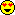## Graph LaplaciansAzimuth

There’s been some new progress on graph Laplacians!

As a mathematical physicist, I’ve always been in love with the Laplacian:

$latex \displaystyle{ \nabla^2 = \frac{\partial^2}{\partial x^2} + \frac{\partial^2}{\partial y^2} + \frac{\partial^2}{\partial z^2} }$It shows up in many of the most fundamental equations of physics: the wave equation, the heat equation, Schrödinger’s equation… and Poisson’s equation:

$latex \nabla^2 \phi = \rho$

which says how the density of matter, $latex \rho,$ affects the gravitational potential $latex \phi.$

As I’ve grown interested in network theory, I’ve gotten more and more interested in ‘graph Laplacians’. These are discretized versions of the Laplacian, where we replace 3-dimensional space by a ‘graph’, meaning something like this:

You can get a lot of interesting information about a graph from its Laplacian. You can also set up discretized versions of all the famous equations I mentioned.

The new progress is a

View original post 540 more words